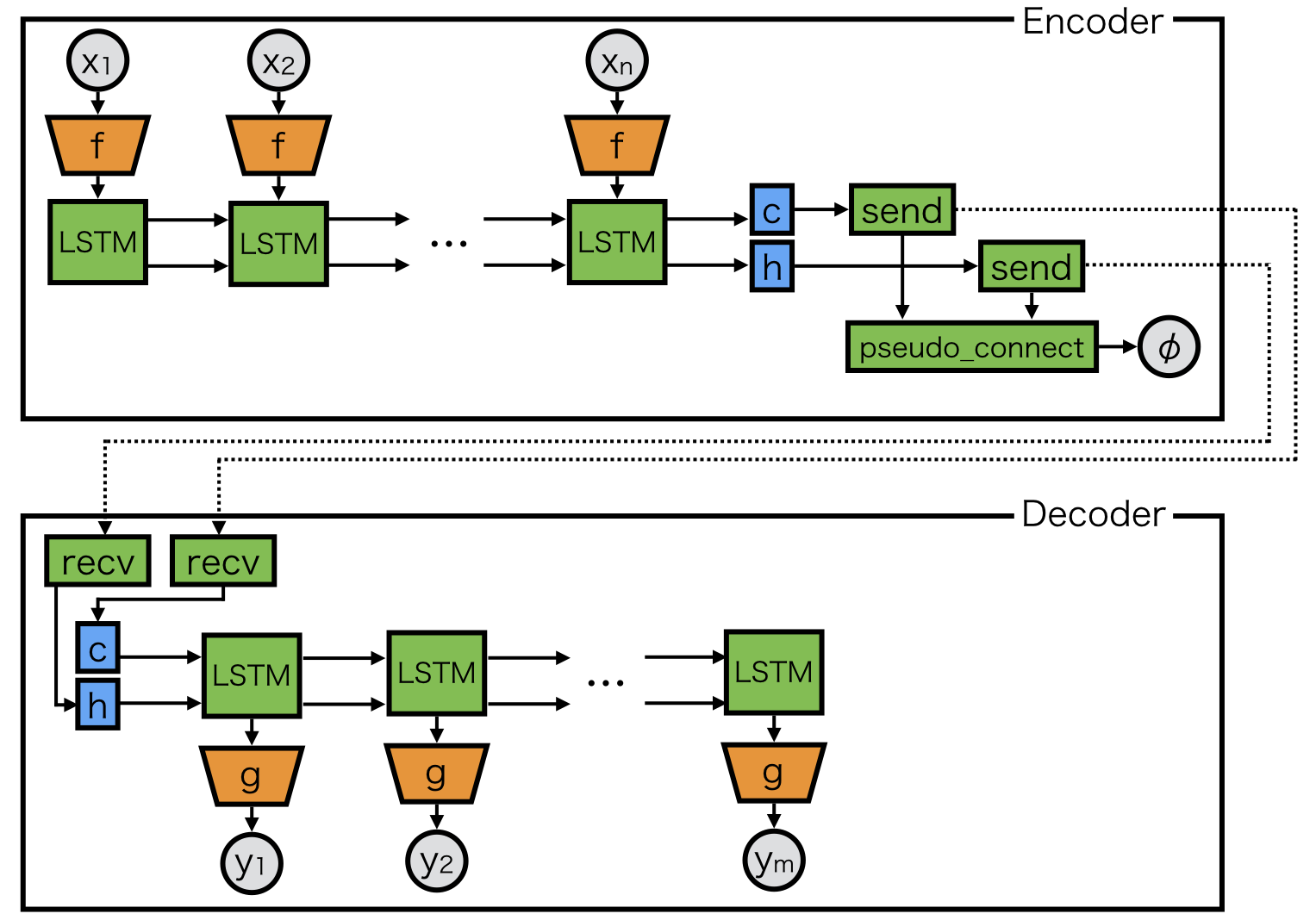# Example 2: seq2seq¶

This example shows how to parallelize models that involves RNN.Above figure depicts a typical encoder-decoder model, where the model is split up to encoder and decoder, both running respectively in two processes. When f or g are large models that consume huge memory such as CNN, model parallelism like this would be useful. In the forward computation, the encoder invokes send function to send its context vectors, and the decoder invokes recv to receive them. The backward computation must be built by pseudo_connect. As this communication pattern is very popular in RNNs, MultiNodeNStepRNN is a ready-made utility link for this pattern. It can replace this complicated communication pattern.

MultiNodeNStepRNN can be created by create_multi_node_n_step_rnn:

rnn = chainermn.links.create_multi_node_n_step_rnn(
L.NStepLSTM(n_layers, n_units, n_units, 0.1),
comm, rank_in=None, rank_out=1)


where comm is a ChainerMN communicator (see Step 1: Communicators).

The overall model definition can be written as follows:

class Encoder(chainer.Chain):

def __init__(self, comm, n_layers, n_units):
super(Encoder, self).__init__(
# Corresponding decoder LSTM will be invoked on process 1.
L.NStepLSTM(n_layers, n_units, n_units, 0.1),
comm, rank_in=None, rank_out=1
),
)
self.comm = comm
self.n_layers = n_layers
self.n_units = n_units

def __call__(self, *xs):
exs = f(xs)
c, h, _, phi = self.mn_encoder(exs)
return phi

class Decoder(chainer.Chain):

def __init__(self, comm, n_layers, n_units):
super(Decoder, self).__init__(
# Corresponding encoder LSTM will be invoked on process 0.